## RS Aggarwal Solutions Class 10 Chapter 9 Mean, Median, Mode of Grouped Data, Cumulative Frequency Graph and O give Ex 9a

These Solutions are part of RS Aggarwal Solutions Class 10. Here we have given RS Aggarwal Solutions Class 10 Chapter 9 Mean, Median, Mode of Grouped Data,Cumulative Frequency Graph and O give Ex 9a.

Other Exercises

Question 1.
Solution:Question 2.
Solution:Question 3.
Solution:Question 4.
Solution:
We haveQuestion 5.
Solution:
We have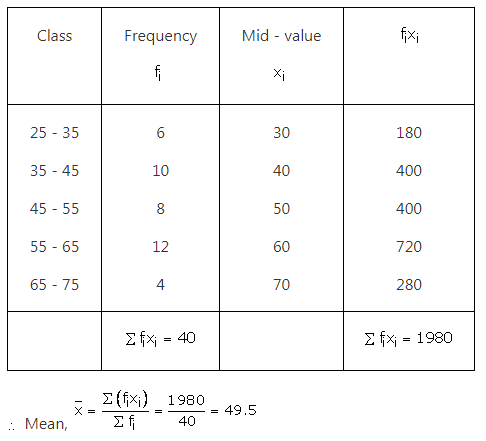Question 6.
Solution:
We haveQuestion 7.
Solution:Question 8.
Solution:Question 9.
Solution:Question 10.
Solution:Question 11.
Solution:Question 12.
Solution:Question 13.
Solution:
We have
7+f1+12+f2+8+5=50 ⇒ f2= 18-f1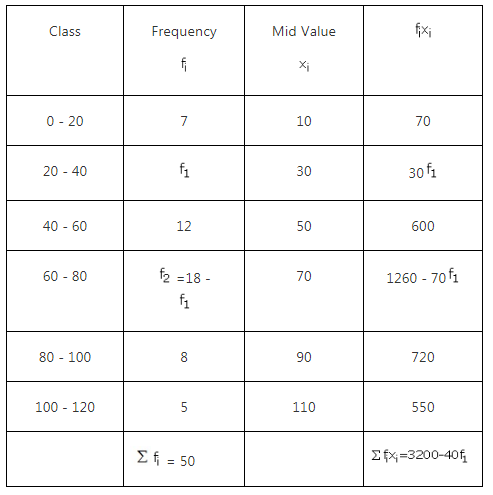Question 14.
Solution:Question 15.
Solution:
We have, Let A = 25 be the assumed mean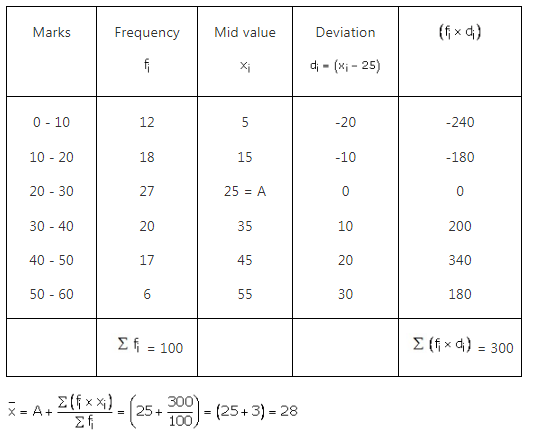Hence mean = 28.

Question 16.
Solution:
Let the assumed mean be 150, h = 20Hence, Mean = 146.25.

Question 17.
Solution:
Let A = 50 be the assumed mean, we have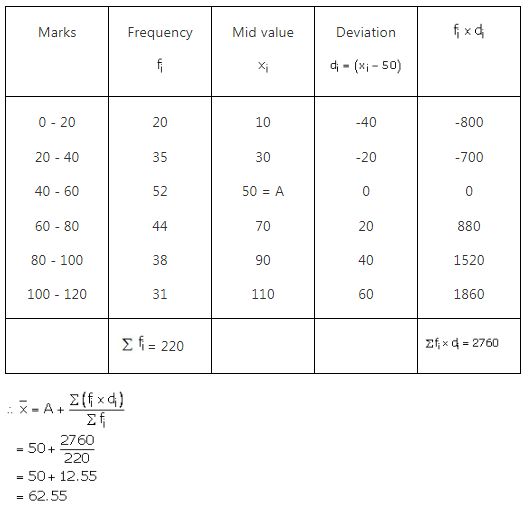Question 18.
Solution:Question 19.
Solution:Question 20.
Solution:Question 21.
Solution:Question 22.
Solution:Question 23.
Solution:Question 24.
Solution:Question 25.
Solution:
Let h = 20 and assume mean = 550, we prepare the table given below: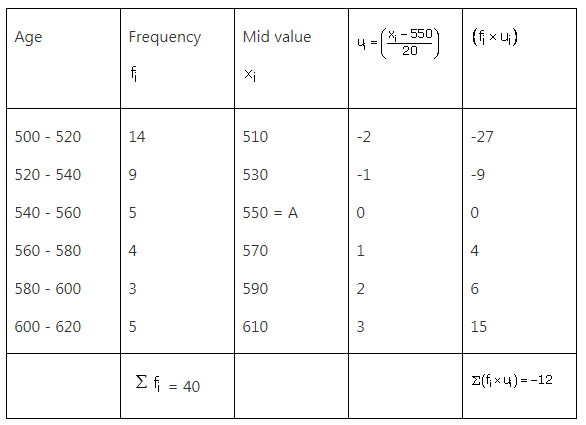Hence the mean of the frequency distribution is 544.

Question 26.
Solution:
The given series is an inclusive series, making it an exclusive series, we have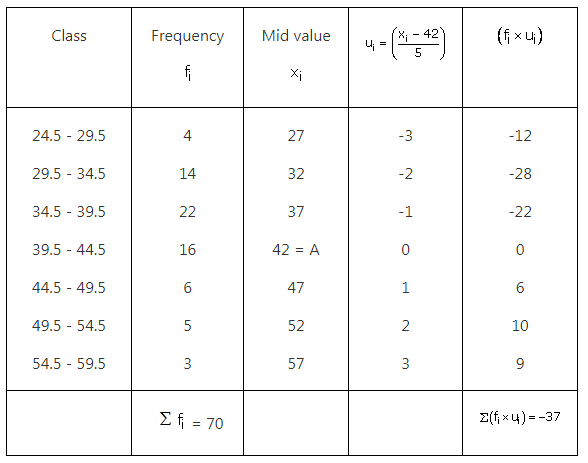Question 27.
Solution:
The given series is an inclusive series making it an exclusive series,we get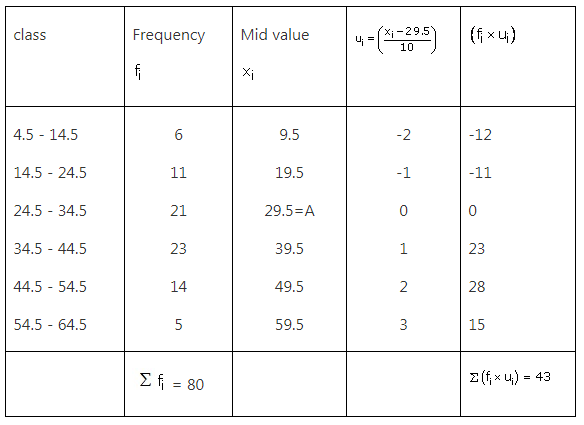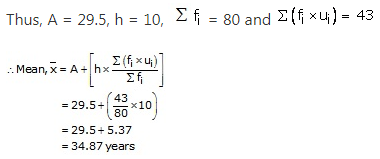Hence, Mean = 34.87 years.

Question 28.
Solution:Question 29.
Solution:We hope the RS Aggarwal Solutions Class 10 Chapter 9 Mean, Median, Mode of Grouped Data Ex 9a help you. If you have any query regarding RS Aggarwal Solutions Class 10 Chapter 9 Mean, Median, Mode of Grouped Data Ex 9a, drop a comment below and we will get back to you at the earliest